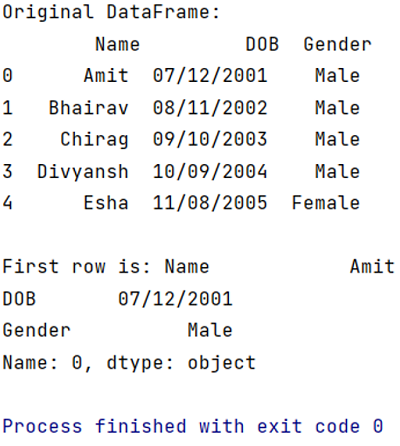# Get First Row of a Pandas DataFrame

Get First Row of DataFrame: In this tutorial, we will learn how can we get the first row value of a given Pandas DataFrame using Python program? By Pranit Sharma Last updated : April 19, 2023

## Get the First Row of Pandas using iloc[]

To get first row of a given Pandas DataFrame, you can simply use the DataFrame.iloc[] property by specifying the row index as 0. Selecting the first row means selecting the index 0. So, we need to pass 0 as an index inside the iloc[] property.

### Syntax

The following code snippet shows to get the first row of a Pandas DataFrame:

```first_row = df.iloc
```

Let us understand with the help of an example.

## Python Program to Get First Row of a Pandas DataFrame

```# Importing pandas package
import pandas as pd

# Creating a Dictionary
dict = {
'Name':['Amit','Bhairav','Chirag','Divyansh','Esha'],
'DOB':['07/12/2001','08/11/2002','09/10/2003','10/09/2004','11/08/2005'],
'Gender':['Male','Male','Male','Male','Female']
}

# Creating a DataFrame
df = pd.DataFrame(dict)

# Display original DataFrame
print("Original DataFrame:\n",df,"\n")

# Selecting First row
first_row = df.iloc

# Display first row
print("First row is:",first_row)
```

### Output

The output of the above program is: